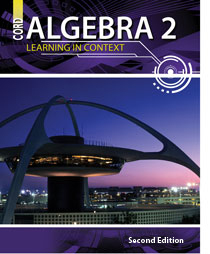# Algebra 2 - 2nd Edition

## Chapter 11: Probability and Statistics

Some links are repeated for use with more than one lesson.

### The following links are applicable to the entire chapter.

http://www.shodor.org/interactivate/activities/expprobability/
This page contains an interactive applet on probability. This website requires Java to run some of its features.

http://www.mathsisfun.com/data/index.html#stats
This collection of lessons on probability covers measures of central tendency and spread of data, and the various types of events measured with probability.

http://www.regentsprep.org/regents/math/algebra/math-ALGEBRA.htm#Probability
The probability and statistics strand of this web site provides links to lessons and practice covering the topics of our chapter, many of which are linked individually below.

### 11.1 Fundamental Theorem of Counting

http://www.regentsprep.org/Regents/math/ALGEBRA/APR1/indexAPR1.htm

http://www.mathsisfun.com/data/basic-counting-principle.html

### 11.2 Compound Events

http://www.regentsprep.org/Regents/math/ALGEBRA/APR8/indexAPR8.htm
This page contains lessons and practice for analyzing compound events involving AND & OR.

http://www.explorelearning.com/index.cfm?method=cSearch.actDoSearch&NewSearch=1&uncompiledQuery=compound+events
This page contains gizmos on compound events. This website requires Shockwave Player to run some of its features.

### 11.3 Collecting Data

http://www.wyzant.com/resources/lessons/math/statistics_and_probability/introduction/sampling
This page presents a variety of probability and non-probability sampling techniques and strategies.

http://www.onlinemathlearning.com/samples-in-probability.html
This page provides several video tutorials addressing topics of importance regarding collecting data, such as sample space.

https://www.math.duke.edu/education/postcalc/probability/prob2.html
This page explores the sample space for simple experiments, and includes a link to an applet to simulate the rolling of dice. This website requires Java to run some of its features.

### 11.4 Probability Models

http://www.math.uah.edu/stat/applets/ConditionalProbabilityExperiment.html
This page presents an applet that demonstrates theoretical and experimental conditional probability. This website requires Java to run some of its features.

http://www.regentsprep.org/regents/math/algtrig/ATS6/indexATS6.htm
This page includes links to examples and practice for comparing expected and observed outcomes, given various conditions.

### 11.5 The Normal Distribution

http://www.regentsprep.org/regents/math/algtrig/ATS2/indexATS2.htm

http://www.mathsisfun.com/data/standard-normal-distribution-table.html
This page includes a dynamic graph that provides percentages for various Z-values for the standard normal
distributions, and a demonstration for how to achieve more accuracy with the normal Z-table. This website requires Java to run some of its features.